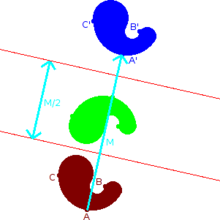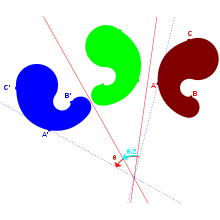# Reflection (mathematics)

Reflection (mathematics)A reflection through an axis followed by a reflection across a second axis parallel to the first one results in a total motion which is a translation.

In mathematics, a reflection (also spelled reflexion) is a mapping from a Euclidean space to itself that is an isometry with a hyperplane as set of fixed points; this set is called the axis (in dimension 2) or plane (in dimension 3) of reflection. The image of a figure by a reflection is its mirror image in the axis or plane of reflection. For example the mirror image of the small Latin letter p for a reflection with respect to a vertical axis would look like q. Its image by reflection in a horizontal axis would look like b. A reflection is an involution: when applied twice in succession, every point returns to its original location, and every geometrical object is restored to its original state.

The term "reflection" is sometimes used for a larger class of mappings from a Euclidean space to itself, namely the non-identity isometries that are involutions. Such isometries have a set of fixed points (the "mirror") that is an affine subspace, but is possibly smaller than a hyperplane. For instance a reflection through a point is an involutive isometry with just one fixed point; the image of the letter p under it would look like a d. This operation is also known as a central inversion (Coxeter 1969, §7.2), and exhibits Euclidean space as a symmetric space. In a Euclidean vector space, the reflection in the point situated at the origin is the same as vector negation. Other examples include reflections in a line in three dimensional space. Typically, however, unqualified use of the term "reflection" means reflection in a hyperplane.

A figure which does not change upon undergoing a reflection is said to have reflectional symmetry.

## Construction

In plane (or 3-dimensional) geometry, to find the reflection of a point one drops a perpendicular from the point onto the line (plane) used for reflection, and continues it to the same distance on the other side. To find the reflection of a figure, one reflects each point in the figure.

## PropertiesA reflection across an axis followed by a reflection in a second axis not parallel to the first one results in a total motion that is a rotation around the point of intersection of the axes.

The matrix for a reflection is orthogonal with determinant -1 and eigenvalues (1, 1, 1, ... 1, -1). The product of two such matrices is a special orthogonal matrix which represents a rotation. Every rotation is the result of reflecting in an even number of reflections in hyperplanes through the origin, and every improper rotation is the result of reflecting in an odd number. Thus reflections generate the orthogonal group, and this result is known as the Cartan–Dieudonné theorem.

Similarly the Euclidean group, which consists of all isometries of Euclidean space, is generated by reflections in affine hyperplanes. In general, a group generated by reflections in affine hyperplanes is known as a reflection group. The finite groups generated in this way are examples of Coxeter groups.

## Reflection across a line in the plane

Reflection across a line through the origin in two dimensions can be described by the following formula$\mathrm{Ref}_l(v) = 2\frac{v\cdot l}{l\cdot l}l - v$

Where v denotes the vector being reflected, l denotes the line being reflected in, and v·l denotes the dot product of v with l. Note the formula above can also be described as$\mathrm{Ref}_l(v) = 2\mathrm{Proj}_l(v) - v\,$

Where the reflection of line l on a is equal to 2 times the projection of v on line l minus v. Reflections in a line have the eigenvalues of 1, and −1.

## Reflection through a hyperplane in n dimensions

Given a vector a in Euclidean space Rn, the formula for the reflection in the hyperplane through the origin, orthogonal to a, is given by$\mathrm{Ref}_a(v) = v - 2\frac{v\cdot a}{a\cdot a}a$

where v·a denotes the dot product of v with a. Note that the second term in the above equation is just twice the vector projection of v onto a. One can easily check that

• Refa(v) = − v, if v is parallel to a, and
• Refa(v) = v, if v is perpendicular to a.

Using the geometric product the formula is a little simpler$\mathrm{Ref}_a(v) = -\frac{a v a}{a^2}$

Since these reflections are isometries of Euclidean space fixing the origin, they may be represented by orthogonal matrices. The orthogonal matrix corresponding to the above reflection is the matrix whose entries are$R_{ij} = \delta_{ij} - 2\frac{a_i a_j}{\|a\|^2}$

where δij is the Kronecker delta.

The formula for the reflection in the affine hyperplane$v\cdot a = c$ not through the origin is$\mathrm{Ref}_{a,c}(v) = v - 2\frac{v\cdot a - c}{a\cdot a}a.$

Wikimedia Foundation. 2010.

### Look at other dictionaries:

• Reflection (linear algebra) — In linear algebra, a reflection is a linear transformation that squares to the identity ( R 2 = I , where R is in K dimensional space), also known as an involution in the general linear group. In addition to reflections across hyperplanes, the… …   Wikipedia

• Reflection seismology — (or seismic reflection) is a method of exploration geophysics that uses the principles of seismology to estimate the properties of the Earth s subsurface from reflected seismic waves. The method requires a controlled seismic source of energy,… …   Wikipedia

• Reflection — or reflexion may refer to:Computers* in computer graphics, the techniques for simulating optical Reflection. * Reflection, a programming language feature for metaprogramming * Reflection , a piece of installation art by Shane Cooper also called… …   Wikipedia

• Reflection group — A reflection group is a group action, acting on a finite dimensional vector space, which is generated by reflections: elements that fix a hyperplane in space pointwise.For example, with regard to ordinary reflections in planes in 3D, a reflection …   Wikipedia

• Reflection principle — This article is about the reflection principles in set theory. For the reflection principle of complex analysis, see Schwarz reflection principleIn set theory, a branch of mathematics, a reflection principle says that it is possible to find sets… …   Wikipedia

• Reflection formula — In mathematics, a reflection formula or reflection relation for a function f is a relationship between f ( a x ) and f ( x ). It is a special case of a functional equation, and it is very common in the literature to refer to use the term… …   Wikipedia

• mathematics — /math euh mat iks/, n. 1. (used with a sing. v.) the systematic treatment of magnitude, relationships between figures and forms, and relations between quantities expressed symbolically. 2. (used with a sing. or pl. v.) mathematical procedures,… …   Universalium

• Mathematics and Physical Sciences — ▪ 2003 Introduction Mathematics       Mathematics in 2002 was marked by two discoveries in number theory. The first may have practical implications; the second satisfied a 150 year old curiosity.       Computer scientist Manindra Agrawal of the… …   Universalium

• Mathematics of Sudoku — The class of Sudoku puzzles consists of a partially completed row column grid of cells partitioned into N regions each of size N cells, to be filled in using a prescribed set of N distinct symbols (typically the numbers {1, ..., N}), so that each …   Wikipedia

• reflection — noun 1》 the fact or phenomenon of light, heat, sound, etc. being reflected.     ↘something reflected or an image so formed. 2》 a thing bringing discredit. 3》 serious thought or consideration.     ↘a considered idea, expressed in writing or speech …   English new terms dictionary